# RS Aggarwal Class 7 Solutions Chapter 21 Collection and Organisation of Data Ex 21A

## RS Aggarwal Class 7 Solutions Chapter 21 Collection and Organisation of Data (Mean, Median and Mode) Ex 21A

These Solutions are part of RS Aggarwal Solutions Class 7. Here we have given RS Aggarwal Solutions Class 7 Chapter 21 Collection and Organisation of Data Ex 21A.

Other Exercises

Question 1.
Solution:
(i) Data : A collection of numerical figures giving some particular type of information is called data
(ii) Raw data : Data obtained in the original form is called raw data.
(iii) Array : Arranging the numerical figures of a data in ascending or descending order is called an array.
(iv) Tabulation of data : Arranging the data in a systematic form in the form of a table is called tabulation of the data.
(v) Observations : Each numerical figure in a data is called an observation.
(vi) Frequency of an observation : The number of times a particular observation occurs is called its frequency.
(vii) Statistics : It is the subject that deals with the collection presentation analysis and interpretation of numerical data.

Question 2.
Solution:
Arranging the given data in ascending order is as given below :
1, 1, 2, 2, 2, 2, 2, 3, 4, 4, 4, 4, 5, 5, 5, 5, 5, 5, 6, 6 and 6 its frequency table will be as under: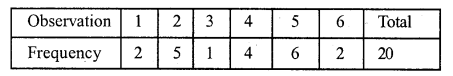Question 3.
Solution:
Arranging the given data in ascending order,
260, 260, 300, 300, 300, 300, 360, 360, 360, 360, 360, 360, 400, 400, 400.
and its frequency table will be as under.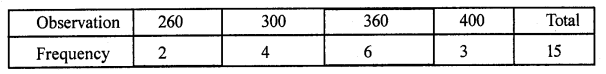Question 4.
Solution:
Arranging the given data in ascending order we find
5, 5, 6, 6, 6, 6, 7, 7, 7, 7, 7, 7, 7, 8, 8, 8, 8, 8, 9, 9, 10, 10 and its frequency table will be as under.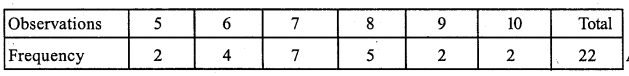Question 5.
Solution:
(i) Data means information in the form of numerical figures.
(ii) Data obtained in the original form is called raw data.
(iii) Arranging the numerical figures in ascending or descending order is called an array.
(iv) The number of times a particular observation occurs is called its frequency.
(v) Arranging the data in the form of a table is called tabulation of data.

Question 6.
Solution:
First five natural numbers are 1, 2, 3, 4, 5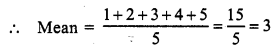Question 7.
Solution:
First six odd natural numbers are 1, 3, 5, 7, 9, 11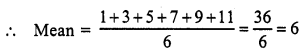Question 8.
Solution:
First seven even natural numbers are 2, 4, 6, 8, 10, 12, 14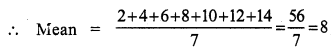Question 9.
Solution:
First five prime numbers are 2, 3, 5, 7, 11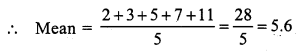Question 10.
Solution:
First six multiples of 5 are 5, 10, 15, 20, 25, 30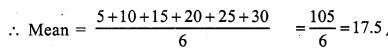Question 11.
Solution: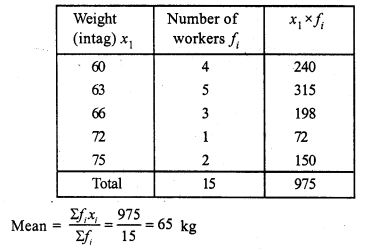Question 12.
Solution: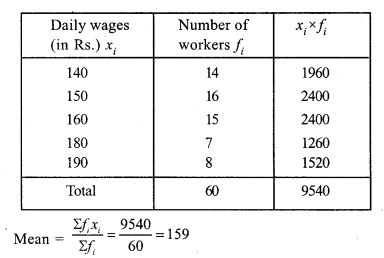Mean = Rs. 159

Question 13.
Solution: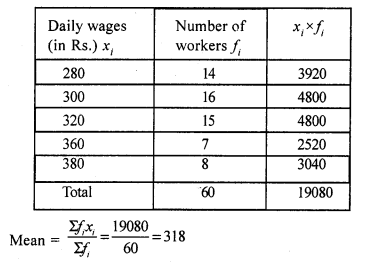Mean = Rs. 318

Question 14.
Solution: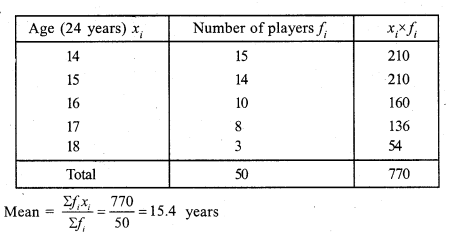Question 15.
Solution: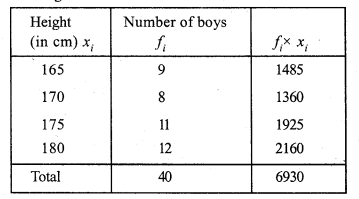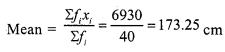Hope given RS Aggarwal Solutions Class 7 Chapter 21 Collection and Organisation of Data Ex 21A are helpful to complete your math homework.

If you have any doubts, please comment below. Learn Insta try to provide online math tutoring for you.